# Code generation¶

Warning

This section is a work in progress, documenting the most recent code generation framework, which is in the package `brian.experimental.codegen2`.

## Overview¶

To generate code, we start with a basic statement or set of statements we want to evaluate for all neurons, or for all synapses, and then apply various transformations to generate code that will do this. We start from a structured, language-invariant representation of the set of basic statements. We then ‘resolve’ the unknown symbols in it. This is done recursively, the resolution of each symbol can add vectorised statements or loops to the current representation, and add data to a namespace that will be associated to the final code. Symbols will be things like a `NeuronGroup` state variable, or a synaptic weight value. The output of this process is a new, more complicated structured representation, including things like loops if necessary. Next, we convert this structured representation into a code string. Finally, this code string is JIT-compiled into an executable object.

### Using numerical integration generation¶

You can use Brian’s equations format to generate C/C++ code for a numerical integration step, for example:

```eqs = '''
dv/dt = (ge+gi-(v+49*mV))/(20*ms) : volt
dge/dt = -ge/(5*ms) : volt
dgi/dt = -gi/(10*ms) : volt
'''
code, vars, params = make_c_integrator(eqs, method=euler, dt=0.1*ms)
print code
```

has output:

```double _temp_v = 50.0*ge + 50.0*gi - 50.0*v - 2.45;
double _temp_ge = -200.0*ge;
double _temp_gi = -100.0*gi;
v += _temp_v*0.0001;
ge += _temp_ge*0.0001;
gi += _temp_gi*0.0001;
```

See the documentation for the function `make_c_integrator()`.

### Using the code generation package¶

The basic way to use the code generation module is as follows:

1. Create a `Block` of `Statement` objects which you want to execute. You can use `statements_from_codestring()` to do this.
2. Create a dictionary of `Symbol` objects corresponding to the symbols in the block above.
3. Call `CodeItem.generate()` with the specified language and symbols, to give you a `Code` object.
4. Optionally, insert additional data into the namespace of the `Code` object.
5. Use the `Code` object via `code(name1=val1, name2=val2)` where the `name=val` are to be inserted into the namespace before the code is called.

This process is very clearly illustrated in the source code for `CodeGenStateUpdater`.

### Structure of the package¶

The following are the main elements of the code generation package:

`Code`
This is the output of the code generation package, a compilable/compiled code fragment, along with a namespace in which it is executed.
`Language`
Used to specify which language the output should have.
`CodeItem`
Before code is converted into a specific language, it is stored in a language-invariant format consisting of `CodeItem` objects, which can in turn contain other `CodeItem` objects. The main derived classes from this are `Block` and `Statement`. The first can contain a series of statements, or it can be a for loop, an if block, etc. A `Statement` can be a `MathematicalStatement` or `CodeStatement`. The former is for things like `x=y*z` and the latter for things like `x = arr[index]`.
`Symbol`, `resolve()`
A `CodeItem` with unresolved dependencies needs to be resolved by the function `resolve()`. Each unresolved depdendency should correspond to a `Symbol` which knows how to modify a `CodeItem` in order to resolve itself. For example, a `NeuronGroupStateVariableSymbol` will insert the `NeuronGroup` state variable value into the namespace, create a new array symbol like `__arr_V` for symbol `V`, and resolve itself either by doing nothing (in Python, as the variable is already vectorised), or by introducing a loop (in C++), or by setting the index variable as the kernel thread (for GPU). For more details, see the section on resolution below.
`make_integration_step()`, `euler()`, `rk2()`, `exp_euler()`
Numerical integration schemes, each integration scheme (such as `euler()`) converts a set of differential equations into a sequence of `MathematicalStatement` objects comprising an integration step.
`CodeGenStateUpdater`, `CodeGenThreshold`, `CodeGenReset`, `CodeGenConnection`
Brian objects using code generation.

## Resolution process¶

### Example¶

```V = V+1
```

Here `V` is a `NeuronGroup` state variable. We wish to transform this into code that can be executed. In the case of Python, the output would look like:

```_neuron_index = slice(None)
V = _arr_V[_neuron_index]
_arr_V[_neuron_index] = V+1
```

The symbol `_arr_V` would be added directly to the namespace.

In the case of C++ it would look like:

```for(int _neuron_index=0; _neuron_index<_len__arr_V; _neuron_index++)
{
double &V = _arr_V[_neuron_index];
V = V+1;
}
```

Here the symbols `_arr_V` and _len__arr_V` would be added to the namespace. The reason for these complicated names is to do with making the code as generic as possible, not introducing namespace clashes (symbols starting with `_` are reserved), etc.

The way the process works is we start with the statement `V=V+1` and a `Symbol` object with name `V`, specifically a `NeuronGroupStateVariableSymbol`. The statement `V=V+1` depends on `V` with both a `Read` and `Write` dependency. We therefore have to ‘resolve’ the symbol `V`. To do this we call the method `resolve()` on `V`.

In the case of Python, this gives us:

```V = _arr_V[_neuron_index]
_arr_V[_neuron_index] = V+1
```

It adds `_arr_V` to the namespace, and creates a dependency on `_neuron_index`. The reason that `V=V+1` is translated to `_arr_V[_neuron_index] = V+1` is that on the left hand side we have a write variable, and on the right hand side we have a read variable. In Python, when vectorising, we have no choice but to give the underlying array with its slice when writing to an array. However, at this point the code generation framework doesn’t know what `_neuron_index` will be, so it could be, for example, an array of indices. In this case, suppose we did `V*V` it would be more efficient to compute `V=_arr_V[_neuron_index]` and then compute `V*V` than to compute `_arr_V[_neuron_index]*_arr_V[_neuron_index]`, and in the case where `_neuron_index=slice(None)` it is no slower, so we always do this.

In the case of C++, the first resolution step gives us:

```double &V = _arr_V[_neuron_index];
V = V+1;
```

For the second resolution step, we need to resolve `_neuron_index`, which is a symbol of type `SliceIndex`, telling us that `_neuron_index` varies over all neurons. Note that we could also have `_neuron_index` being an `ArrayIndex`, for examples `spikes`, and then this could be used for a reset operation (we would iterate only over those indices of neurons which had spiked). Here though, we iterate over all neurons. In Python, calling the `resolve()` method of `_neuron_index` gives us:

```_neuron_index = slice(None)
V = _arr_V[_neuron_index]
_arr_V[_neuron_index] = V+1
```

and in C++:

```for(int _neuron_index=0; _neuron_index<_len__arr_V; _neuron_index++)
{
double &V = _arr_V[_neuron_index];
V = V+1;
}
```

In both cases, the `_neuron_index` symbol is resolved and the process is complete.

Note that we have actually mixed two stages here, the stage of generating a structured representation of the code using `CodeItem` objects, and the stage of generating code strings using `CodeItem.convert_to()`. In fact, the converting of, for example, `V` to `_arr_V[_neuron_index]` only happens at the second stage.

### `resolve()`¶

The first stage, acting on the structured representation of nested `CodeItem` objects is resolved using the function `resolve()`. This calls `Symbol.resolve()` for each of the symbols in turn. The resolution order is determined by an optimal efficiency algorithm, see the reference documentation for `resolve()` for the full algorithm description.

`Symbol.resolve()` can do an arbitrary transformation of the input `CodeItem`, but typically it will either do something like:

```load()
item
save()
```

Or something like:

```for name in array:
item
```

See the reference documentation for `Symbol.resolve()`, and the documentation for the most important symbols:

### `convert_to()`¶

This step is relatively straightforward, each `CodeItem` object has its `convert_to` method called iteratively. The important one is in `MathematicalStatement`, where the left hand side usage is replaced by `Symbol.write()` and the right hand side usage is replaced by `Symbol.read()`. In addition, at this stage the syntax of mathematical statements is corrected, e.g. Python’s `x**y` is replaced by C++’s `pow(x,y)` using `sympy`.

## Code generation in Brian¶

The four objects used for code generation in Brian are:

`CodeGenStateUpdater`
Used for numerical integration, see above and reference documentation.
`CodeGenThreshold`
Used for computing a threshold function.
`CodeGenReset`
Used for computing post-spike reset.
`CodeGenConnection`
Used for synaptic propagation.

### Numerical integration¶

An integration scheme is generated from an `Equations` object using the `make_integration_step()` function. See reference documentation for that function for details.

This is carried out by `CodeGenStateUpdater`, which can be used as a Brian `brian.StateUpdater` object in `brian.NeuronGroup`.

As an example, for Euler integration, the differential equations:

```dx/dt = expr
```

are separated by `Equations` into variable `x` with expression `expr`. This then becomes:

```_temp_x := expr
x += _temp_x*dt
```

This can then be resolved by the code generation mechanisms described already.

### Synaptic propagation¶

TODO: synaptic propagation, including docstrings and code comments

NOTE: GPU functionality not included for synaptic propagation yet.

## GPU¶

Warning

GPU code is highly transitional, many details may change in the future.

GPU code is handled by five classes:

`GPULanguage` (derived from `CLanguage`)
Identifies the language as CUDA, and stores a singleton `GPUManager` object which is used to manage the GPU.
`GPUCode` (derived from `Code`)
Returned from the code generation process, but mostly just acts as a proxy to `GPUManager`.
`GPUKernel`
Handles the final stage of taking a partially generated kernel (without the vectorisation over threads) and computing the final kernel (using vectorisation over threads). Also adds data to the `GPUSymbolMemoryManager`.
`GPUManager`
Manages the GPU generally. Stores a set of kernels (`GPUKernel`) and manages memory via `GPUSymbolMemoryManager`. Handles joining the memory management code and kernel code into a single source file, and compiling it.
`GPUSymbolMemoryManager`
Handles allocation of GPU memory for symbols.

For more details, see the reference documentation for the classes in the order above.

Note that `CodeGenConnection` is the only code generation version of a Brian class which is not GPU enabled at present.

## Extending code generation¶

To extend code generation, you will probably need to add new `Symbol` classes. Read the documentation for this class to start, and the documentation for the most important symbols:

See also `CodeItem`, particularly the process described in `CodeItem.generate()`.

## Inheritance diagrams¶

The overall structure of the classes in the code generation package are included below, for reference.

### Languages¶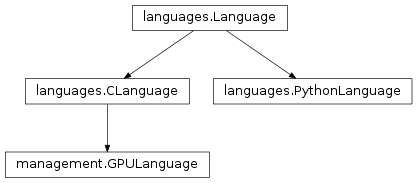### Code objects¶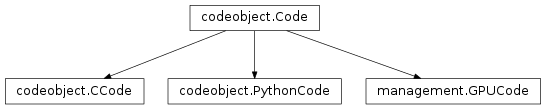### Code items¶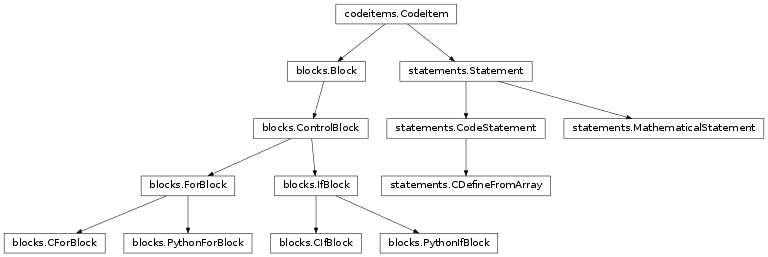### Equations¶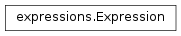### Symbols¶### Resolution and code output¶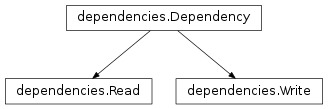### Integration¶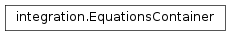### GPU¶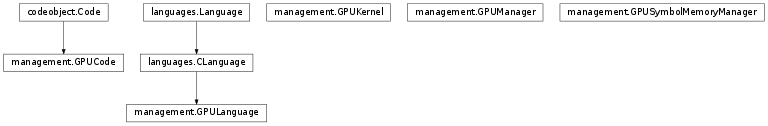### Brian objects¶

#### Connection¶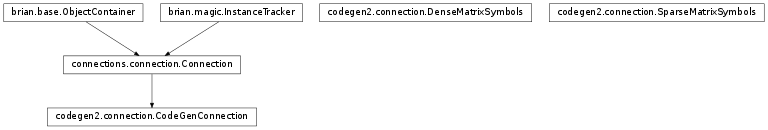#### Reset¶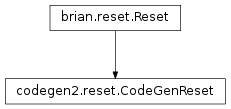#### State updater¶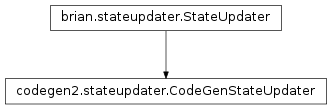#### Threshold¶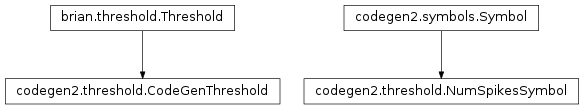## Reference¶

### blocks¶

class `brian.experimental.codegen2.``Block`(*args)

Contains a list of `CodeItem` objects which are considered to be executed in serial order. The list is passed as arguments to the init method, so if you want to pass a list you can initialise as:

```block = Block(*items)
```
class `brian.experimental.codegen2.``ControlBlock`(start, end, contents, dependencies, resolved)

Helper class used as the base for various control structures such as for loops, if statements. These are typically not language-invariant and should only be output in the resolution process by symbols (which know the language they are resolving to). Consists of strings `start` and `end`, a list of `contents` (as for `Block`), and explicit sets of `dependencies` and `resolved` (these are self-dependencies/resolved). The output code consists of the start string, the indented converted contents, and then the end string. For example, for a C for loop, we would have `start='for(...){` and `end='}'`.

`convert_to`(language, symbols={}, namespace={})
class `brian.experimental.codegen2.``ForBlock`(start, end, contents, dependencies, resolved)

Simply a base class, does nothing.

class `brian.experimental.codegen2.``PythonForBlock`(var, container, content, dependencies=None, resolved=None)

A for loop in Python, the structure is:

```for var in container:
content
```

Where `var` and `container` are strings, and `content` is a `CodeItem` or list of items.

Dependencies can be given explicitly, or by default they are `Read(x)` for each word `x` in `container`. Resolved can be given explicitly, or by default it is `set(var)`.

class `brian.experimental.codegen2.``CForBlock`(var, spec, content, dependencies=None, resolved=None)

A for loop in C, the structure is:

```for(spec)
{
content
}
```

You specify a string `var` which is the variable the loop is iterating over, and a string `spec` should be of the form `'int i=0; i<n; i++'`. The `content` is a `CodeItem` or list of items. The dependencies and resolved sets can be given explicitly, or by default they are extracted, respectively, from the set of words in `spec`, and `set([var])`.

class `brian.experimental.codegen2.``IfBlock`(start, end, contents, dependencies, resolved)

Just a base class.

class `brian.experimental.codegen2.``PythonIfBlock`(cond, content, dependencies=None, resolved=None)

If statement in Python, structure is:

```if cond:
content
```

Dependencies can be specified explicitly, or are automatically extracted as the words in string `cond`, and resolved can be specified explicitly or by default is `set()`.

class `brian.experimental.codegen2.``CIfBlock`(cond, content, dependencies=None, resolved=None)

If statement in C, structure is:

```if(cond)
{
content
}
```

Dependencies can be specified explicitly, or are automatically extracted as the words in string `cond`, and resolved can be specified explicitly or by default is `set()`.

### codeitems¶

class `brian.experimental.codegen2.``CodeItem`

An item of code, can be anything from a single statement corresponding to a single line of code, right up to a block with nested loops, etc.

Should define the following attributes (default values are provided):

`resolved`
The set of dependencies which have been resolved in this item, including in items contained within this item. Default value: the union of `selfresolved` and `subresolved`. Elements of the set should be of type `Dependency` (i.e. `Read` or `Write`).
`selfresolved`
The set of dependencies resolved only in this item, and not in subitems. Default value: `set()`.
`subresolved`
The set of dependencies resolved in subitems, default value is the union of `item.dependencies` for each `item` in this item. Requires the `CodeItem` to have an iterator, i.e. a method `__iter__`.
`dependencies`, `selfdependencies`, `subdependencies`
As above for resolved, but giving the set of dependencies in this code. The default value for `dependencies` takes the union of `selfdependencies` and `subdependencies` and removes all the symbols in `resolved`.

This structure of having default implementations allows several routes to derive a class from here, e.g.:

`Block`
Simply defines a list attribute `contents` which is a sequence of items, and implements `__iter__` to return `iter(contents)`.
`CodeStatement`
Defines a fixed string which is not language-invariant, and a fixed set of dependencies and resolved. The `convert_to()` method simply returns the fixed string. Does not define an `__iter__` method because the default values for `dependencies` and `resolved` are overwritten.
`convert_to`(language, symbols={}, namespace={})

Returns a string representation of the code for this item in the given language. From the user point of view, you should call `generate()`, but in developing new `CodeItem` derived classes you need to implement this. The default behaviour is simply to concatenate the strings returned by the subitems.

`generate`(name, language, symbols, namespace=None)

Returns a `Code` object. The method resolves the symbols using `resolve()`, converts to a string with `convert_to()` and then converts that to a `Code` object with `Language.code_object()`.

`subdependencies`
`subresolved`

### codeobject¶

class `brian.experimental.codegen2.``Code`(name, code_str, namespace, pre_code=None, post_code=None, language=None)

The basic Code object used for all Python/C/GPU code generation.

The Code object has the following attributes:

`name`
The name of the code, should be unique. This matters particularly for GPU code which uses the name attribute for the kernel function names.
`code_str`
A representation of the code in string form
`namespace`
A dictionary of name/value pairs in which the code will be executed
`code_compiled`
An optional value (can be None) consisting of some representation of the compiled form of the code
`pre_code`, `post_code`
Two optional Code objects which can be in the same or different languages, and can share partially or wholly the namespace. They are called (respectively) before or after the current code object is executed.
`language`
A `Language` object that stores some global settings and state for all code in that language.

Each language (e.g. PythonCode) extends some or all of the methods:

`__init__(...)`
Unsurprisingly used for initialising the object, should call Code.__init__ with all of its arguments.
`compile()`
Compiles the code, if necessary. If not necessary, set the `code_compiled` value to any dummy value other than None.
`run()`
Runs the compiled code in the namespace.

It will usually not be necessary to override the call mechanism:

`__call__(**kwds)`
Calls `pre_code(**kwds)`, updates the namespace with `kwds`, executes the code (calls `self.run()`) and then calls `post_code(**kwds)`.
`compile`()
`run`()
class `brian.experimental.codegen2.``PythonCode`(name, code_str, namespace, pre_code=None, post_code=None, language=None)
`compile`()
`run`()
class `brian.experimental.codegen2.``CCode`(name, code_str, namespace, pre_code=None, post_code=None, language=None)
`run`()

### connection¶

class `brian.experimental.codegen2.``CodeGenConnection`(*args, **kwds)
`propagate`(spikes)
class `brian.experimental.codegen2.``DenseMatrixSymbols`
class `SynapseIndex`(M, name, weightname, language, sourceindex='_source_index', targetlen='_target_len')
`resolve`(read, write, vectorisable, item, namespace)
class `TargetIndex`(M, name, weightname, language, index='_synapse_index', targetlen='_target_len')
`dependencies`()
`load`(read, write, vectorisable)
`supported_languages` = ['python', 'c']
class `Value`(M, name, language, index='_synapse_index')
class `brian.experimental.codegen2.``SparseMatrixSymbols`
class `SynapseIndex`(M, name, weightname, language, sourceindex='_source_index')
`resolve`(read, write, vectorisable, item, namespace)
class `TargetIndex`(M, name, weightname, language, index='_synapse_index')
class `Value`(M, name, language, index='_synapse_index')

### dependencies¶

class `brian.experimental.codegen2.``Dependency`(name)

Base class for `Read` and `Write` dependencies.

A dependency marks that a `CodeItem` depends on a given symbol. Each dependency has a `name`.

class `brian.experimental.codegen2.``Read`(name)

Used to indicate a read dependency, i.e. the value of the symbol is read.

class `brian.experimental.codegen2.``Write`(name)

Used to indicate a write dependency, i.e. the value of the symbol is written to.

`brian.experimental.codegen2.``get_read_or_write_dependencies`(dependencies)

Returns the set of names of the variables which are either read to or written to in a set of dependencies.

### equations¶

`brian.experimental.codegen2.``freeze_with_equations`(inputcode, eqs, ns)

Returns a frozen version of `inputcode` with equations and namespace.

Replaces each occurrence in `inputcode` of a variable name in the namespace `ns` with its value if it is of int or float type. Variables with names in `brian.Equations` `eqs` are not replaced, and neither are `dt` or `t`.

`brian.experimental.codegen2.``frozen_equations`(eqs)

Returns a frozen set of equations.

Each expression defining an equation is frozen as in `freeze_with_equations()`.

### expressions¶

class `brian.experimental.codegen2.``Expression`(expr)

A mathematical expression such as `x*y+z`.

Has an attribute `dependencies` which is `Read(var)` for all words `var` in `expr`.

Has a method `convert_to()` defined the same way as `CodeItem.convert_to()`.

`convert_to`(language, symbols={}, namespace={})

Converts expression into a string for the given `language` using the given set of `symbols`. Replaces each `Symbol` appearing in the expression with `sym.read()`, and if the language is C++ or GPU then uses `sympy.CCodePrinter().doprint()` to convert the syntax, e.g. `x**y` becomes `pow(x,y)`.

### formatting¶

`brian.experimental.codegen2.``word_substitute`(expr, substitutions)

Applies a dict of word substitutions.

The dict `substitutions` consists of pairs `(word, rep)` where each word `word` appearing in `expr` is replaced by `rep`. Here a ‘word’ means anything matching the regexp `\bword\b`.

`brian.experimental.codegen2.``flattened_docstring`(docstr, numtabs=0, spacespertab=4, split=False)

Returns a docstring with the indentation removed according to the Python standard

split=True returns the output as a list of lines

Changing numtabs adds a custom indentation afterwards

`brian.experimental.codegen2.``indent_string`(s, numtabs=1, spacespertab=4, split=False)

Indents a given string or list of lines

split=True returns the output as a list of lines

`brian.experimental.codegen2.``get_identifiers`(expr)

Return all the identifiers in a given string `expr`, that is everything that matches a programming language variable like expression, which is here implemented as the regexp `\b[A-Za-z_][A-Za-z0-9_]*\b`.

`brian.experimental.codegen2.``strip_empty_lines`(s)

Removes all empty lines from the multi-line string `s`.

### gpu¶

Warning

GPU code is highly transitional, many details may change in the future.

class `brian.experimental.codegen2.``GPUKernel`(name, code, namespace, mem_man, maxblocksize=512, scalar='double', force_sync=True)

Generates final kernel source code and used to launch kernels.

Used in conjunction with `GPUManager`. Each kernel is prepared with `prepare()` which generates source code and adds symbols to the `GPUSymbolMemoryManager`. The `GPUManager` compiles the code and sets the `gpu_func` attribute, and the kernel can then be called via `run()`.

The initialisation method extracts variable `_gpu_vector_index` from the namespace and stores it as attribute `index`, and `_gpu_vector_slice` as the pair `(start, end)`.

`prepare`()

Generates kernel source code and adds symbols to memory manager.

We extract the number of GPU indices from the namespace, `_num_gpu_indices`.

We loop through the namespace, and for each value determine it to be either an array or a single value. If it is an array, then we place it in the `GPUSymbolMemoryManager`, otherwise we add it to the list of arguments provided to the function call. This allows scalar variables like `t` to be transmitted to the kernel function in its arguments.

We then generate a kernel of the following (Python template) form:

```__global__ void {name}({funcargs})
{{
int {vector_index} = blockIdx.x * blockDim.x + threadIdx.x;
if(({vector_index}<({start}))||({vector_index}>=({end})))
return;
{code_str}
}}
```

We also compute the block size and grid size using the user provided maximum block size.

`prepare_gpu_func`()

Calls the `pycuda` GPU function `prepare()` method for low-overhead function calls.

`run`()

Calls the function on the GPU, extracting the scalar variables in the argument list from the namespace.

class `brian.experimental.codegen2.``GPUManager`(force_sync=True, usefloat=False)

This object controls everything on the GPU.

It uses a `GPUKernel` object for managing kernels, and a `GPUSymbolMemoryManager` object for managing symbol memory.

The class is used by:

1. Adding several kernels using `add_kernel()`
2. Calling `prepare()` (see method documentation for details)
3. Run code with `run()`

Memory is mirrored on GPU and CPU. In the present implementation, in the development phase only, each call to `run()` will copy all symbols from CPU to GPU before running the GPU kernel, and afterwards copy all symbols from GPU back to CPU. In the future, this will be disabled and symbol memory copies will be handled explicitly by calls to methods `copy_to_device()` and `copy_to_host()`.

`add_kernel`(name, code, namespace)

Adds a kernel with the given name, code and namespace. Creates a `GPUKernel` object.

`add_symbols`(items)
`compile`()

Compiles code using `pycuda.compiler.SourceModule` and extracts kernel functions with `pycuda.compiler.SourceModule.get_function()`. The `GPUKernel.gpu_func` attribute is set for each kernel.

`copy_to_device`(symname)
`copy_to_host`(symname)
`generate_code`()

Combines kernel source into one source file, and adds memory management kernel functions. These simple kernels simply copy a pointer to a previously specified name. This is necessary because when `pycuda` is used to allocate memory, it doesn’t give it a name only a pointer, and the kernel functions use a named array.

`initialise_memory`()

Copies allocated memory pointers to named global memory pointer variables so that kernels can use them. The kernel names to do this are in the `GPUSymbolMemoryManager.symbol_upload_funcnames` dict (keys are symbol names), and the allocated pointers are in the `GPUSymbolMemoryManager.device` dict.

`make_combined_kernel`(*names)

Not used at present. Will be used to combine multiple kernels with the same vectorisation index for efficiency.

`prepare`()

Compiles code and initialises memory.

Performs the following steps:

1. `GPUKernel.prepare()` is called for each kernel, converting the partial code into a complete kernel, and adds symbols to the `GPUSymbolMemoryManager`, which allocates space on the GPU and copies data to it from the CPU.
2. `generate_code()` is called, combining individual kernels into one source file, and adding memory management kernels and declarations.
3. `compile()` is called, which JIT-compiles the code using `pycuda`.
4. `initialise_memory()` is called, which allocates memory
`run`(name)

Runs the named kernel. Calls `GPUKernel.run()`. Note that all symbols are copied to and from the GPU before and after the kernel run, although this is only for the development phase and will change later.

class `brian.experimental.codegen2.``GPUSymbolMemoryManager`(usefloat=False)

Manages symbol memory on the GPU.

Stores an attribute `device` and `host` which are dicts, with keys the symbol names, and values `pycuda.gpuarray.GPUArray` and `numpy.ndarray` respectively. Add symbols with `add_symbols()`, which will allocate memory.

`add_symbols`(items)

Each item in `items` is of the form `(symname, hostarr, devname)` where `symname` is the symbol name, `hostarr` is the `numpy.ndarray` containing the data, and `devname` is the name the array pointer should have on the device.

Allocates memory on the device, and copies data to the GPU.

`copy_to_device`(symname)

Copy the memory in the `numpy.ndarray` for `symname` to the allocated device memory. If `symname==True`, do this for all symbols. You can also pass a list for `symname`.

`copy_to_host`(symname)

As for `copy_to_device()` but copies memory from device to host.

`generate_code`()

Generates declarations for array pointer names on the device, and kernels to copy device pointers to the array pointers. General form is:

```__device__ {dtypestr} *{name};
__global__ void set_array_{name}({dtypestr} *_{name})
{
{name} = _{name};
}
```

Stores the kernel function names in attribute `symbol_upload_funcnames` (dict with keys being symbol names).

Returns a string with declarations and kernels combined.

`names`

The list of symbol names managed.

class `brian.experimental.codegen2.``GPUCode`(name, code_str, namespace, pre_code=None, post_code=None, language=None)

`Code` object for GPU.

For the user, works as the same as any other `Code` object. Behind the scenes, source code is passed to the `GPUManager` `gpu_man` from the `GPULanguage` object, via `GPUManager.add_kernel()`. Compilation is handled by `GPUManager.prepare()`, and running code by `GPUManager.run()`.

`compile`()

Simply calls `GPUManager.prepare()`.

`run`()

Simply runs the kernel via `GPUManager.run()`.

class `brian.experimental.codegen2.``GPULanguage`(scalar='double', gpu_man=None, force_sync=True)

`Language` object for GPU.

Has an attribute `gpu_man`, the `GPUManager` object responsible for allocating, copying memory, etc. One is created if you do not specify one.

`CodeObjectClass`

alias of `GPUCode`

### integration¶

class `brian.experimental.codegen2.``EquationsContainer`(eqs)

Utility class for defining numerical integration scheme

Initialise with a set of equations `eqs`. You can now iterate over this object in two ways, firstly over all the differential equations:

```for var, expr in eqscontainer:
yield f(expr)
```

Or over just the differential equations with nonzero expressions (i.e. not including `dx/dt=0` for parameters):

```for var, expr in eqscontainer.nonzero:
yield f(expr)
```

Here `var` is the name of the symbol, and `expr` is a string, the right hand side of the differential equation `dvar/dt=expr`.

Also has attributes:

`names`
The symbol names for all the differential equations
`names_nonzero`
The symbol names for all the nonzero differential equations
`brian.experimental.codegen2.``make_integration_step`(method, eqs)

Return an integration step from a method and a set of equations.

The `method` should be a function `method(eqs)` which receives a `EquationsContainer` object as its argument, and `yield` s statements. For example, the `euler()` integration step is defined as:

```def euler(eqs):
for var, expr in eqs.nonzero:
yield '_temp_{var} := {expr}'.format(var=var, expr=expr)
for var, expr in eqs.nonzero:
yield '{var} += _temp_{var}*dt'.format(var=var, expr=expr)
```
`brian.experimental.codegen2.``euler`(eqs)

Euler integration

`brian.experimental.codegen2.``rk2`(eqs)

2nd order Runge-Kutta integration

`brian.experimental.codegen2.``exp_euler`(eqs)

Exponential-Euler integration

### languages¶

class `brian.experimental.codegen2.``Language`(name)

Base class for languages, each should provide a `name` attribute, and a method `code_object()`.

`code_object`(name, code_str, namespace)

Return a `Code` object from a given `name`, code string `code_str` and `namespace`. If the class has a class attribute `CodeObjectClass`, the default implementation returns:

```CodeObjectClass(name, code_str, namespace, language=self)
```
class `brian.experimental.codegen2.``PythonLanguage`

Python language.

`CodeObjectClass`

alias of `PythonCode`

class `brian.experimental.codegen2.``CLanguage`(scalar='double')

C language.

Has an attribute `scalar='double'` which gives the default type of scalar values (used when `dtype` is not specified). This can be used, for example, on the GPU where `double` may not be available.

`CodeObjectClass`

alias of `CCode`

### makeintegrator¶

`brian.experimental.codegen2.``make_c_integrator`(eqs, method, dt, values=None, scalar='double', timeunit=second, timename='t')

Gives C/C++ format code for the integration step of a differential equation.

`eqs`
The equations, can be an `brian.Equations` object or a multiline string in Brian equations format.
`method`
The integration method, typically `euler()`, `rk2()` or `exp_euler()`, although you can pass your own integration method, see `make_integration_step()` for details.
`dt`
The value of the timestep dt (in Brian units, e.g. `0.1*ms`)
`values`
Optional, dictionary of mappings variable->value, these values will be inserted into the generated code.
`scalar`
By default it is `'double'` but if you want to use float as your scalar type, set this to `'float'`.
`timename`
The name of the time variable (if used). In Brian this is ‘t’, but you can change it to ‘T’ or ‘time’ or whatever. This can be used if you want users to specify time in Brian form (‘t’) but the context in which this code will be used (e.g. another simulator) specifies time with a different variable name (e.g. ‘T’).
`timeunit`
The unit of the time variable, scaled because Brian expects time to be in seconds.

Returns a triple `(code, vars, params)`:

`code`
The C/C++ code to perform the update step (string).
`vars`
A list of variable names.
`params`
A list of per-neuron parameter names.

### reset¶

class `brian.experimental.codegen2.``CodeGenReset`(group, inputcode, language, level=0)

### resolution¶

`brian.experimental.codegen2.``resolve`(item, symbols, namespace=None)

Resolves `symbols` in `item` in the optimal order.

The first stage of this algorithm is to construct a dependency graph on the symbols.

The optimal order is resolve loops as late as possible. We actually construct the inverse of the resolution order, which is the intuitive order (i.e. if the first thing we do is loop over a variable, then that variable is the last symbol we resolve).

We start by finding the set of symbols which have no dependencies. The graph is acyclic so this always possible. Then, among those candidates, if possible we choose loopless symbols first (this corresponds to executing loops as late as possible). With this symbol removed from the graph we repeat until all symbols are placed in order.

We then resolve in reverse order (because we start with the inner loop and add code outwards). At the beginning of this stage, vectorisable is set to `True`. But after we encounter the first multi-valued symbol we set `vectorisable` to `False` (we can only vectorise one loop, and it has to be the innermost one). This vectorisation is used by both Python and GPU but not C++. Each resolution step calls `CodeItem.resolve()` on the output of the previous stage.

### statements¶

class `brian.experimental.codegen2.``Statement`

Just a base class, supposed to indicate single line statements.

class `brian.experimental.codegen2.``CodeStatement`(code, dependencies, resolved)

A language-specific single line of code, which should only be used in the resolution step by a `Symbol` which knows the language it is resolving to. The string `code` and the set of `dependencies` and `resolved` have to be given explicitly.

`convert_to`(language, symbols={}, namespace={})
class `brian.experimental.codegen2.``CDefineFromArray`(var, arr, index, dependencies=None, resolved=None, dtype=None, reference=True, const=False)

Define a variable from an array and an index in C.

For example:

```double &V = __arr_V[neuron_index];
```

Initialisation arguments are:

`var`
The variable being defined, a string.
`arr`
A string representing the array.
`index`
A string giving the index.
`dependencies`
Given explicitly, or by default use `set([Read(arr), Read(index)])`.
`resolved`
Given explicitly, or by default use `set([var])`.
`dtype`
The numpy data type of the variable being defined.
`reference`
Whether the variable should be treated as a C++ reference (e.g. `double &V = ...` rather than `double V = ...`. If the variable is being written to as well as read from, use `reference=True`.
`const`
Whether the variable can be defined as const, specify this if only reading the value and not writing to it.
class `brian.experimental.codegen2.``MathematicalStatement`(var, op, expr, dtype=None)

A single line mathematical statement.

The structure is `var op expr`.

`var`
The left hand side of the statement, the value being written to, a string.
`op`
The operation, can be any of the standard Python operators (including `+=` etc.) or a special operator `:=` which means you are defining a new symbol (whereas `=` means you are setting the value of an existing symbol).
`expr`
A string or an `Expression` object, giving the right hand side of the statement.
`dtype`
If you are defining a new variable, you need to specify its numpy dtype.

If `op==':='` then this statement will resolve `var`, otherwise it will add a `Write` dependency for `var`. The other dependencies come from `expr`.

`convert_to`(language, symbols={}, tabs=0, namespace={})

When converting to a code string, the following takes place:

• If the LHS variable is in the set of `symbols`, then the LHS is replaced by `sym.write()`
• The expression is converted with `Expression.convert_to()`.
• If the operation is definition `op==':='` then the output is language dependent. For Python it is `lhs = rhs` and for C or GPU it is `dtype lhs = rhs`.
• If the operation is not definition, the statement is converted to `lhs op rhs`.
• If the language is C/GPU the statement has `;` appended.
`brian.experimental.codegen2.``statements_from_codestring`(code, eqs=None, defined=None, infer_definitions=False)

Generate a list of statements from a user-defined string.

`code`
The input code string, a multi-line string which should be flat, no indents.
`eqs`
A Brian `Equations` object, which is used to specify a set of already defined variable names if you are using `infer_definitions`.
`defined`
A set of symbol names which are already defined, if you are using `infer_definitions`.
`infer_definitions`
Set to `True` to guess when a line of the form `a=b` should be inferred to be of type `a:=b`, as user-specified code may not make the distinction between `a=b` and `a:=b`.

The rule for definition inference is that you scan through the lines, and a set of already defined symbols is maintained (starting from `eqs` and `defined` if provided), and an `=` op is changed to `:=` if the name on the LHS is not already in the set of symbols already defined.

`brian.experimental.codegen2.``c_data_type`(dtype)

Gives the C language specifier for numpy data types. For example, `numpy.int32` maps to `int32_t` in C.

Perhaps this method is given somewhere in numpy, but I couldn’t find it.

### stateupdater¶

class `brian.experimental.codegen2.``CodeGenStateUpdater`(group, method, language, clock=None)

State updater using code generation, supports Python, C++, GPU.

Initialised with:

`group`
The `NeuronGroup` that this will be used in.
`method`
The integration method, currently one of `euler()`, `rk2()` or `exp_euler()`, but you can define your own too. See `make_integration_step()` for details.
`language`
The `Language` object.

Creates a `Block` from the equations and the `method`, gets a set of `Symbol` objects from `get_neuron_group_symbols()`, and defines the symbol `_neuron_index` as a `SliceIndex`. Then calls `CodeItem.generate()` to get the `Code` object.

Inserts `t` and `dt` into the namespace, and `_num_neurons` and `_num_gpu_indices` in case they are needed.

### symbols¶

class `brian.experimental.codegen2.``Symbol`(name, language)

Base class for all symbols.

Every symbol has attributes `name` and `language` which should be a string and `Language` object respectively. The symbol class should define some or all of the methods below.

`dependencies`()

Returns the set of dependencies of this symbol, can be overridden.

`load`(read, write, vectorisable)

Called by `resolve()`, can be overridden to perform more complicated loading code. By default, returns an empty `Block`.

`multi_valued`()

Should return `True` if this symbol is considered to have multiple values, for example if you are iterating over an array like so:

```for(int i=0; i<n; i++)
{
double &x = arr[i];
...
}
```

Here the symbol `x` is single-valued and depends on the symbol `i` which is multi-valued and whose resolution required a loop. By default returns `False` unless the class has an attribute `multiple_values` in which case this is returned.

`read`()

The string that should be used when this symbol is read, by default just the symbol name.

`resolution_requires_loop`()

Should return `True` if the resolution of this symbol will require a loop. The `resolve()` function uses this to optimise the symbol resolution order.

`resolve`(read, write, vectorisable, item, namespace)

Creates a modified item in which the symbol has been resolved.

For example, if we started from the expression:

```x += 1
```

and we wanted to produce the following C++ code:

```for(int i=0; i<n; i++)
{
double &x = __arr_x[i];
x += 1;
}
```

we would need to take the expression `x+=1` and embed it inside a loop.

Function arguments:

`read`
Whether or not we read the value of the symbol. This is computed by analysing the dependencies by the main `resolve()` function.
`write`
Whether or not we write a value to the symbol.
`vectorisable`
Whether or not the expression is vectorisable. In Python, we can only vectorise one multi-valued index, so if there are two or more, only the innermost loop will be vectorised.
`item`
The code item which needs to be resolved.
`namespace`
The namespace to put data in.

The default implementation first calls `update_namespace()`, then creates a new `Block` consisting of the value returned by `load()`, the `item`, and the value returned by `save()`. Finally, this symbol’s name is added to the `resolved` set for this block.

`save`(read, write, vectorisable)

Called by `resolve()`, can be overridden to perform more complicated saving code. By default, returns an empty `Block`.

`supported`()

Returns `True` if the language specified at initialisation is supported. By default, checks if the language name is in the class attribute `supported_languages` (list), however can be overridden.

`supported_languages` = []
`update_namespace`(read, write, vectorisable, namespace)

Called by `resolve()`, can be overridden to modify the namespace, e.g. adding data.

`write`()

The string that should be used when this symbol is written, by default just the symbol name.

class `brian.experimental.codegen2.``RuntimeSymbol`(name, language)

This Symbol is guaranteed by the context to be inserted into the namespace at runtime and can be used without modification to the name, for example `t` or `dt`.

`supported`()

Returns `True`.

class `brian.experimental.codegen2.``ArraySymbol`(arr, name, language, index=None, array_name=None)

This symbol is used to specify a value taken from an array.

Schematically: `name = arr[index]`.

`arr` (numpy array)
The numpy array which the values will be taken from.
`name`, `language`
The name of the symbol and language.
`index`
The index name, by default `'_index_'+name`.
`array_name`
The name of the array, by default `'_arr_'+name`.

Introduces a read-dependency on `index` and `array_name`.

`dependencies`()

`load`(*args, **kwds)

Method generated by `language_invariant_symbol_method()`.

Languages and methods follow:

`python`
`load_python()`
`gpu`
`load_c()`
`c`
`load_c()`
`load_c`(read, write, vectorisable)
`load_python`(read, write, vectorisable)

If `read` is false, does nothing. Otherwise, returns a `CodeStatement` of the form:

```name = array_name[index]
```
`supported_languages` = ['python', 'c', 'gpu']
`update_namespace`(read, write, vectorisable, namespace)

Adds pair `(array_name, arr)` to namespace.

`write`(*args, **kwds)

Method generated by `language_invariant_symbol_method()`.

Languages and methods follow:

`python`
`write_python()`
`gpu`
`write_c()`
`c`
`write_c()`
`write_c`()
`write_python`()

Returns `array_name[index]`.

class `brian.experimental.codegen2.``NeuronGroupStateVariableSymbol`(group, varname, name, language, index=None)

Symbol for a state variable.

Wraps `ArraySymbol`.

Arguments:

`name`, `language`
Symbol name and language.
`group`
The `NeuronGroup`.
`varname`
The state variable name in the group.
`index`
An index name (or use default of `ArraySymbol`).
class `brian.experimental.codegen2.``SliceIndex`(name, start, end, language, all=False)

Multi-valued symbol that ranges over a slice.

Schematically: `name = slice(start, end)`

`name`, `language`
Symbol name and language.
`start`
The initial value, can be an integer or string.
`end`
The final value (not included), can be an integer or string.
`all`
Set to `True` to indicate that the slice covers the whole range possible (small optimisation for Python).
`multiple_values` = True
`resolution_requires_loop`()

Returns `True` except for Python.

`resolve`(*args, **kwds)

Method generated by `language_invariant_symbol_method()`.

Languages and methods follow:

`python`
`resolve_python()`
`gpu`
`resolve_gpu()`
`c`
`resolve_c()`
`resolve_c`(read, write, vectorisable, item, namespace)

Returns `item` embedded in a C for loop.

`resolve_gpu`(read, write, vectorisable, item, namespace)

If not `vectorisable` return `resolve_c()`. If `vectorisable` we mark it by adding `_gpu_vector_index = name` and `_gpu_vector_slice = (start, end)` to the namespace. The GPU code will handle this later on.

`resolve_python`(read, write, vectorisable, item, namespace)

If `vectorisable` and `all` then we simply return `item` and add `name=slice(None)` to the namespace.

If `vectorisable` and not `all` then we prepend the following statement to `item`:

```name = slice(start, end)
```

If not `vectorisable` then we add a for loop over `xrange(start, end)`.

`supported_languages` = ['python', 'c', 'gpu']
class `brian.experimental.codegen2.``ArrayIndex`(name, array_name, language, array_len=None, index_name=None, array_slice=None)

Multi-valued symbol giving an index that iterates through an array.

Schematically: `name = array_name[array_slice]`

`name`, `language`
Symbol name and language.
`array_name`
The name of the array we iterate through.
`array_len`
The length of the array (int or string), by default has value `'_len_'+array_name`.
`index_name`
The name of the index into the array, by default has value `'_index_'+array_name`.
`array_slice`
A pair `(start, end)` giving a slice of the array, if left the whole array will be used.

Dependencies are collected from those arguments that are used (`item`, `array_name`, `array_len`, `array_slice`).

`multiple_values` = True
`resolution_requires_loop`()

Returns `True` except for Python.

`resolve`(*args, **kwds)

Method generated by `language_invariant_symbol_method()`.

Languages and methods follow:

`python`
`resolve_python()`
`c`
`resolve_c()`
`resolve_c`(read, write, vectorisable, item, namespace)

Returns a C++ for loop of the form:

```for(int index_name=start; index_name<end; index_name++)
{
const int name = array_name[index_name];
...
}
```

If defined `(start, end)=array_slice` otherwise `(start, end)=(0, array_len)`.

`resolve_gpu`(read, write, vectorisable, item, namespace)

If not vectorisable, use `resolve_c()`. If vectorisable, we set the following in the namespace:

```_gpu_vector_index = index_name
_gpu_vector_slice = (start, end)
```

Where `start` and `end` are as in `resolve_c()`. This marks that we want to vectorise over this index, and the GPU code will handle this later. Finally, we prepend the item with:

const int name = array_name[index_name];
`resolve_python`(read, write, vectorisable, item, namespace)

If vectorisable it will prepend one of these two forms to `item`:

```name = array_name
name = array_name[start:end]
```

(where `(start, end) = array_slice` if provided).

If not vectorisable, it will return a for loop over either `array_name` or array_name[start:end]`.

`supported_languages` = ['python', 'c', 'gpu']
`brian.experimental.codegen2.``language_invariant_symbol_method`(basemethname, langs, fallback=None, doc=None)

Helper function to create methods for `Symbol` classes.

Sometimes it is clearer to write a separate method for each language the `Symbol` supports. This function can generate a method that can take any language, and calls the desired method. For example, if you had defined two methods `load_python` and `load_c` then you would define the `load` method as follows:

```load = language_invariant_symbol_method('load',
```

The `fallback` gives a method to call if no language-specific method was found. A docstring can be provided to `doc`.

`brian.experimental.codegen2.``get_neuron_group_symbols`(group, language, index='_neuron_index', prefix='')

Returns a dict of `NeuronGroupStateVariable` from a group.

Arguments:

`group`
The group to extract symbols from.
`language`
The language to use.
`index`
The name of the neuron index, by default `_neuron_index`.
`prefix`
An optional prefix to add to each symbol.

### threshold¶

class `brian.experimental.codegen2.``CodeGenThreshold`(group, inputcode, language, level=0)